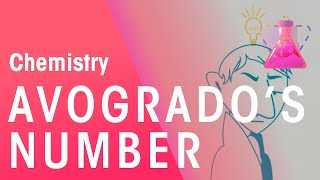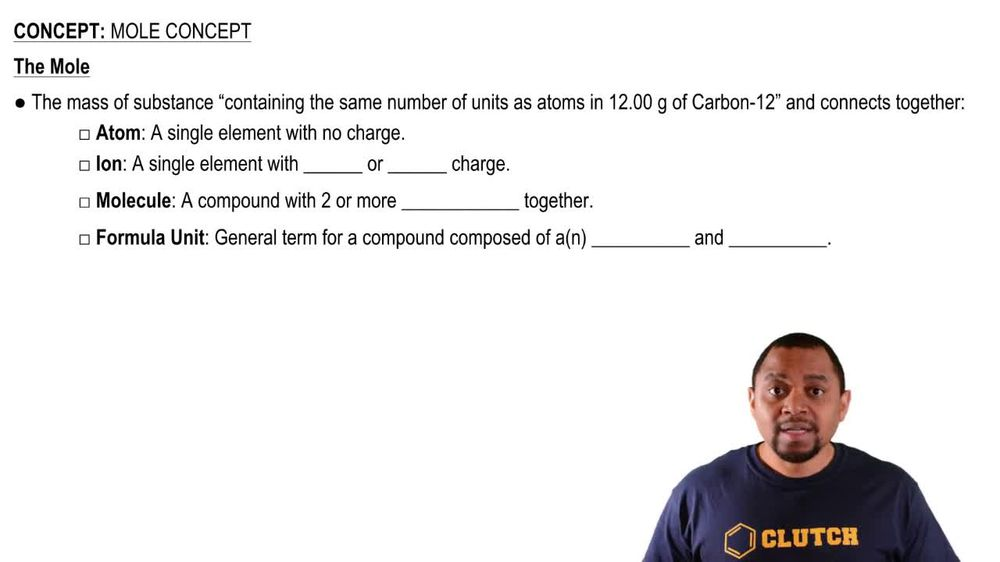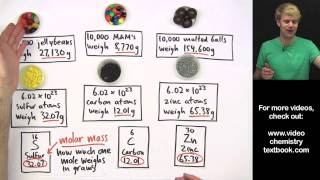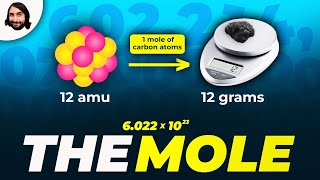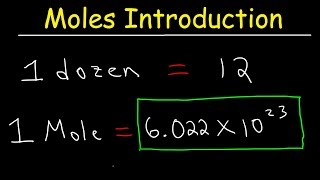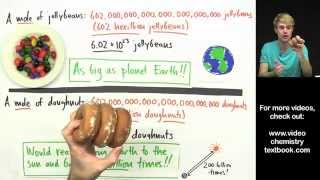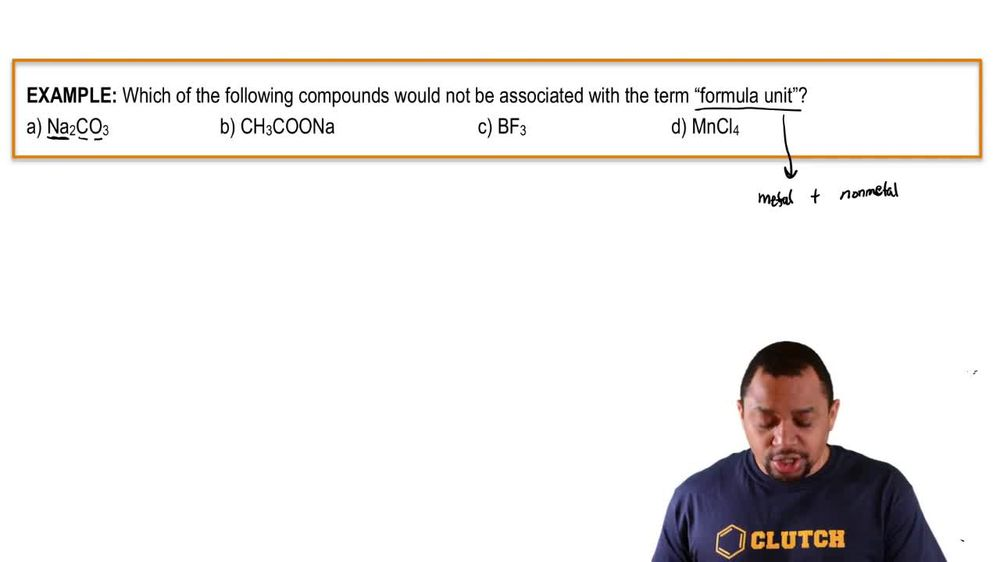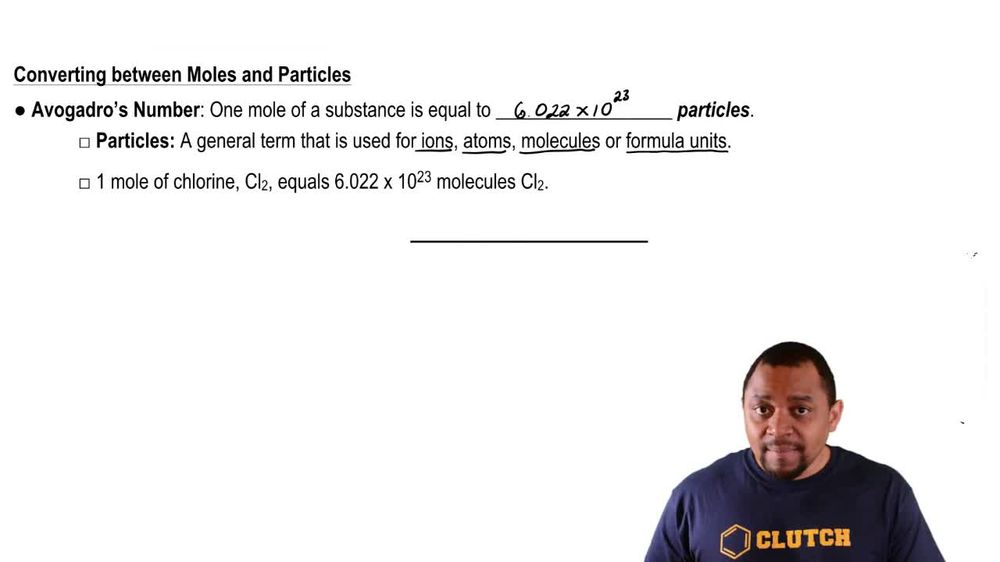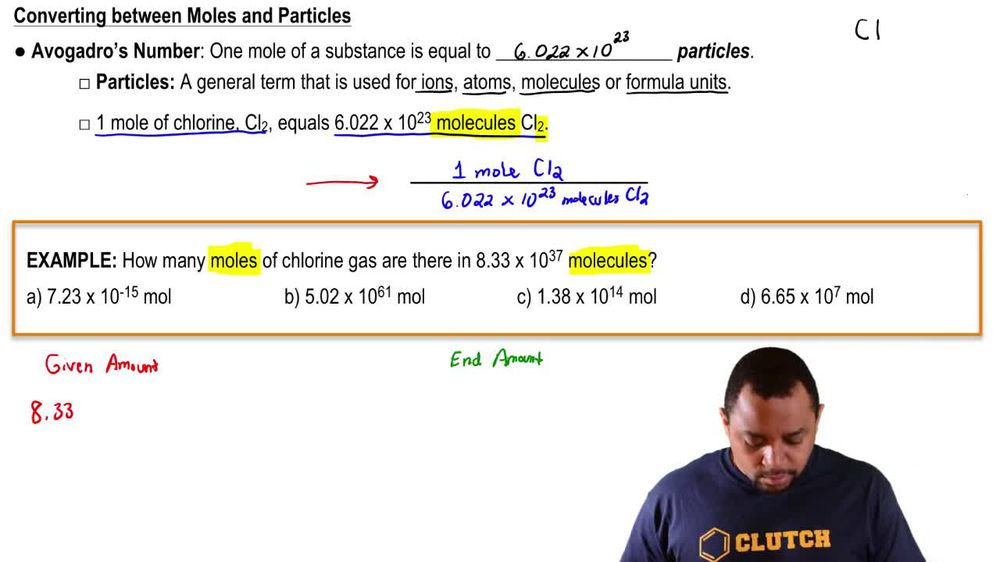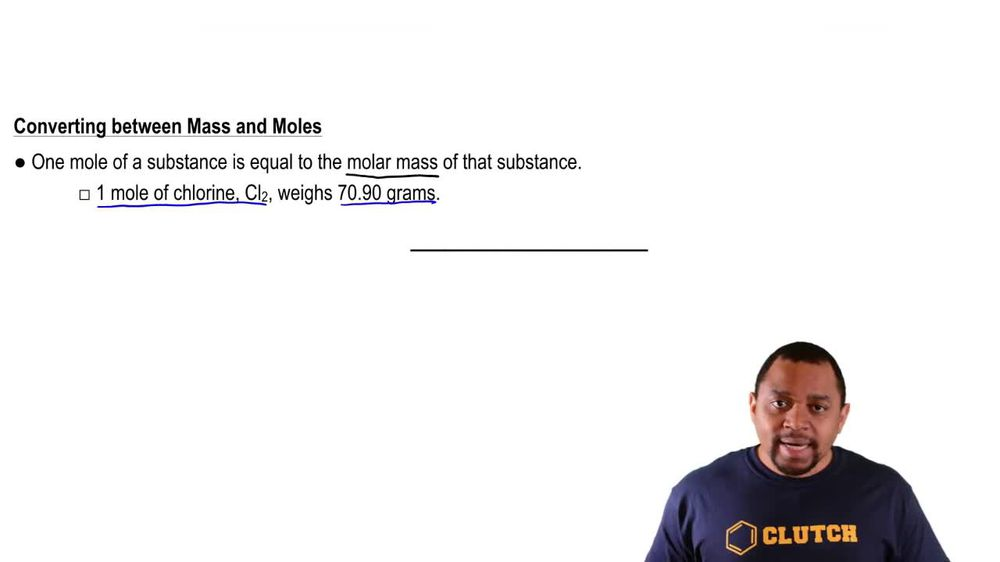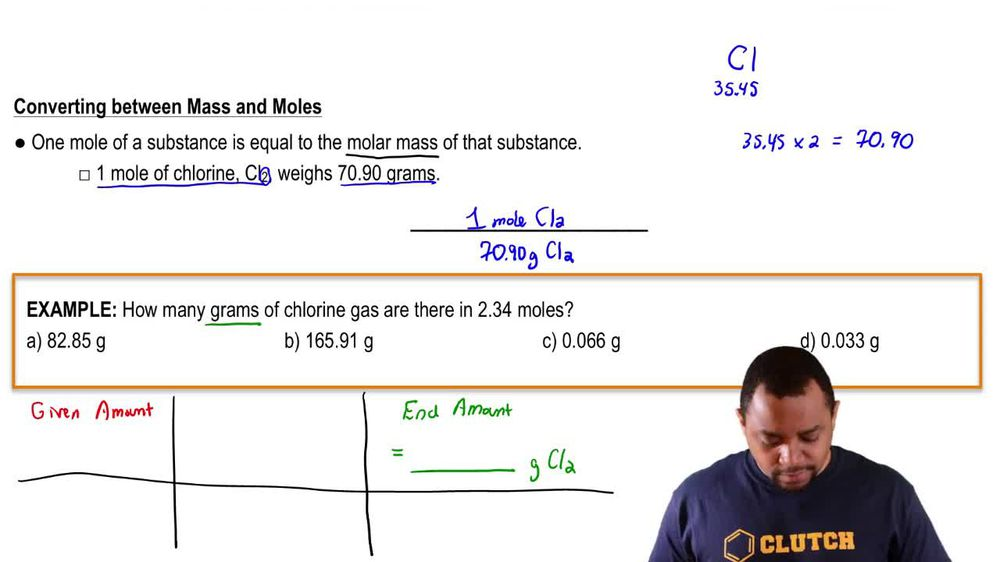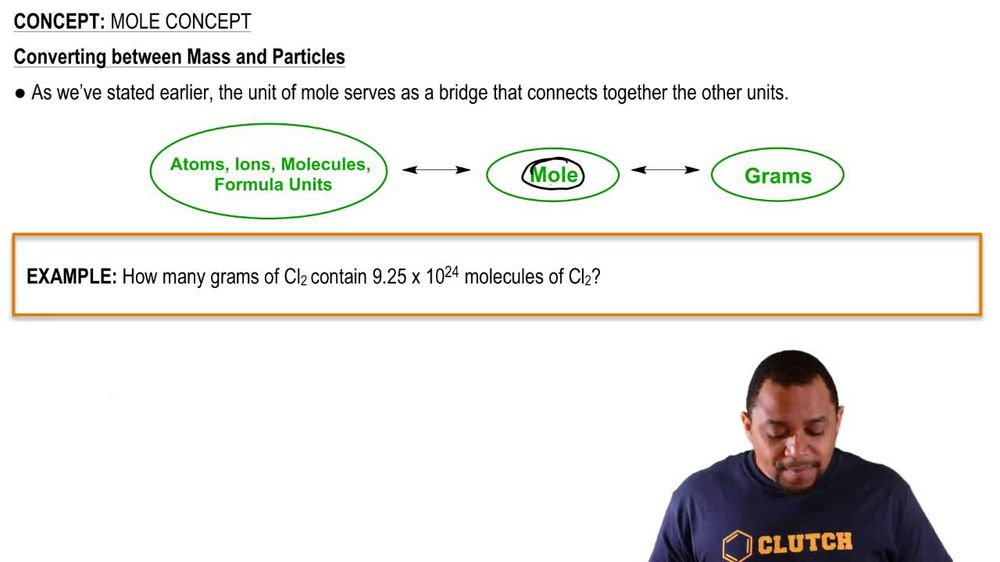Start typing, then use the up and down arrows to select an option from the list.
1. 2. Atoms & Elements2. Mole Concept
Problem

# How many moles are in each of the following samples? (a) 11.51 g of Ti (b) 29.127 g of Na (c) 1.477 kg of U

Relevant Solution3m
Play a video:
Hey everyone welcome back. So let's get started with this video. So here they want us to convert 2.36g of each element into malls. So the way this is going to be possible is to find the molar masses of each element and we can find them on the periodic table. So for calcium We find that it's 40 point 078 g per mole. Okay? And then for zinc we find that it's 65 point 38 grams per mole. And then finally for Krypton that is point 798 grams per mole. And once again we can find these on the periodic table. So then let's start with calcium. So we're going to start with the given. So it is too point g of calcium C. A. They were going to use the molar mass to convert it into moles. And there is 40 point 078g of calcium Per one mole of calcium. So then here the g of calcium will cancel out and we get moles of calcium and then we do the math. And we get zero zero five eight nine moles of calcium. Okay, so then let's go ahead and work with B. Which is zinc. So once again let's start with the given Which is two 36 g of zinc. Then we're going to use a molar mass which we said was 0.38 grams of sink For one more zinc. So then here grams of zinc cancel out We do the math. And we get 0. 361 most of zinc. And then we're going to do it now with C, which is krypton. Once again starting with the same grounds 2. grounds of krypton which is KR we're going to use the molar mass to convert to moles. It's 83.798 g of Krypton. For everyone. More of krypton grounds of krypton cancel that. We get most and that is zero point - 8 to moles. Okay, Thank you for watching. I hope this helped. And I'll see you in the next video.